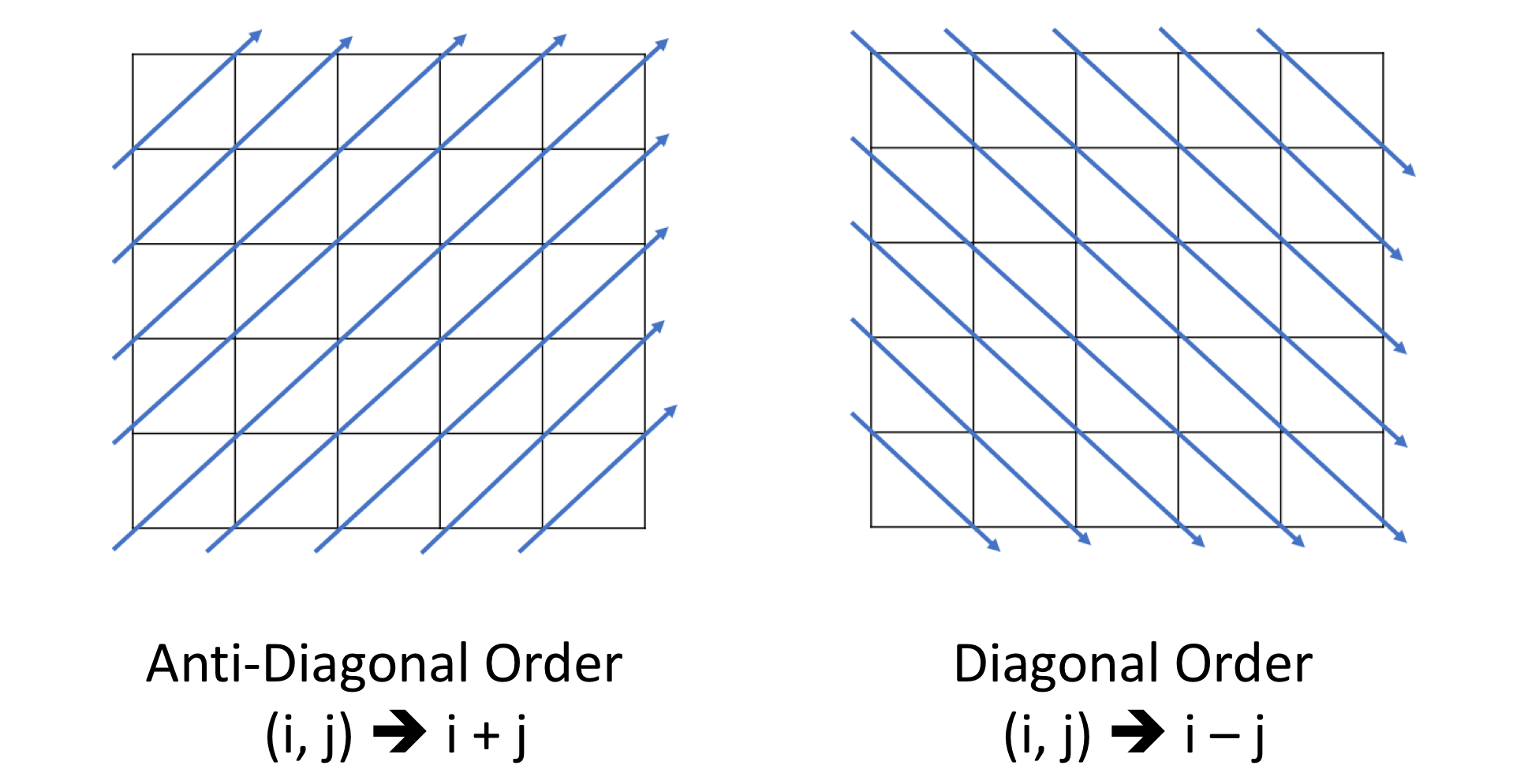# 一、哈希表

## 1、哈希表的原理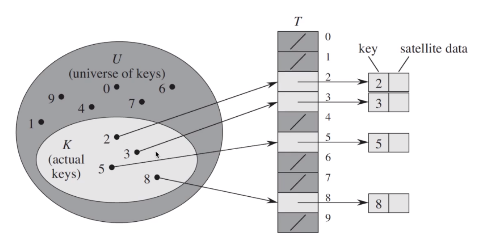$U$为键值的全域，包含了所有可能的键值，$K$为实际使用的键值，$T$为直接寻址表，每个关键字对应表中的一个位置，比如2、3、5、8这几个关键字对应了$T(2),T(3),T(5),T(8)$。$T(K)$则指向了关键字为$K$的元素。但直接寻址有这样的缺点：（1）当$U$很大时，需要消耗大量的内存；（2）若$U$很大而$K$很小，则有很多空间被浪费；（3）无法处理关键字不是数字的情况。为改进这些缺点，可以使用哈希函数。直接寻址表是键值为$K$的元素放到$T(K)$位置上，而哈希函数构建一个大小为$m$的寻址表$T$，键值为$K$的元素放到$h(K)$的位置上。$h(K)$是一个函数，将域$U$映射到表$T[0, 1, …, m-1]$。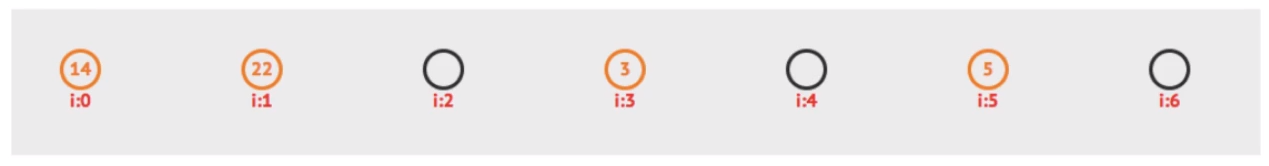14余7等于0，存在了$T(0)$的位置，22余7等于1，存在了$T(1)$的位置。这样做也会有问题，比如再存一个键值为15的元素，15余7等于1，但此时$T(1)$已经被占了，这就是哈希冲突。如果位置$i$被占，可以解决哈希冲突的方法：

• 线性探查：探查$i+1,i+2,…$这些位置
• 二次探查：$i+1^2, i-1^2, i+2^2, i-2^2,…$这些位置
• 二度哈希：有$n$个哈希函数，当第一个哈希函数冲突时，则使用第二个、第三个……
• 拉链法：哈希表每个位置都连一个链表，冲突的元素将被加到该位置链表的最后。如下图所示：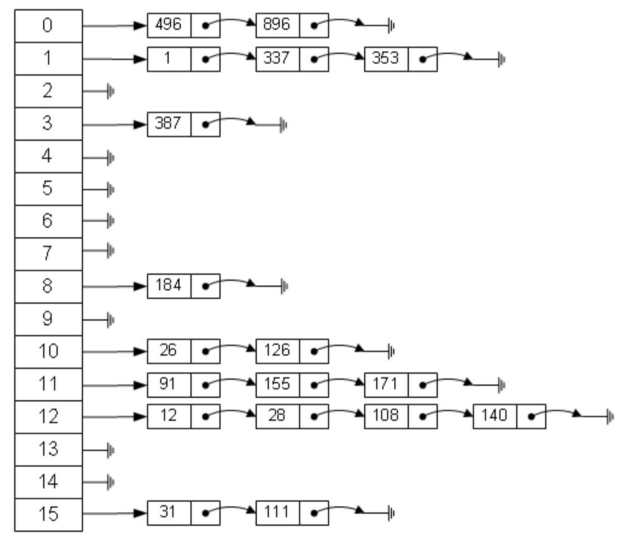常用的哈希函数：

• 除法哈希：$h(K)=K\%m$

• 乘法哈希：$h(K)=floor(m \times (A*K\%1))$
• 全域哈希：$h(K)=((a \times K + b) \% p) \% m \space \space \space \space a,b=1,2,…p-1$

## 2、python里哈希的用法

• 哈希集合是集合数据结构的实现之一，用于存储非重复值。python里可以用集合set实现：
# 1. 初始化哈希集合
hashset = set()
# 2. 添加新的键
# 3. 删除键
hashset.remove(2)
# 4. 检查键是否在哈希集合里
if (2 not in hashset):
print("Key 2 is not in the hash set.")
# 5. 获得哈希集合的大小
print("Size of hashset is:", len(hashset))
# 6. 迭代哈希集合
for x in hashset:
print(x, end=" ")
print("are in the hash set.")
# 7. 清空哈希集合
hashset.clear()
print("Size of hashset:", len(hashset))
• 哈希映射是映射 数据结构的实现之一，用于存储(key, value)键值对。在python可以用字典dict实现：
# 1. 初始化哈希映射
hashmap = {0 : 0, 2 : 3}
# 2. 插入(key, value) 或者更新已存在的键值对
hashmap = 1
hashmap = 2
# 3. 获得键值
print("The value of key 1 is: " + str(hashmap))
# 4. 删除键
del hashmap
# 5. 检查键是否在哈希集合里
if 2 not in hashmap:
print("Key 2 is not in the hash map.")
# 6. 键和键值可以有不同的数据类型
hashmap["pi"] = 3.1415
# 7. 获得哈希映射的大小
print("The size of hash map is: " + str(len(hashmap)))
# 8. 迭代哈希映射
for key in hashmap:
print("(" + str(key) + "," + str(hashmap[key]) + ")", end=" ")
print("are in the hash map.")
# 9. 获得所有键
print(hashmap.keys())
# 10. 清空哈希映射
hashmap.clear();
print("The size of hash map is: " + str(len(hashmap)))

# 二、例题

## 1、哈希集合的应用

def containsDuplicate(self, nums: List[int]) -> bool:
hash_ = set()
for num in nums:
if num not in hash_:
else:
return True
return False

• 时间复杂度 : $O(n)$。
• 空间复杂度 : $O(n)$。哈希表占用的空间与元素数量是线性关系。

class Solution:
def intersection(self, nums1: List[int], nums2: List[int]) -> List[int]:
set1 = set(nums1)
set2 = set(nums2)
return list(set2 & set1)

• 时间复杂度：$O(m+n)$，其中 $n$ 和 $m$ 是数组的长度。$O(n)$ 的时间用于转换 nums1 在集合中，$O(m)$ 的时间用于转换 nums2 到集合中，并且平均情况下，集合的操作为 $O(1)$。
空间复杂度：$O(m+n)$，最坏的情况是数组中的所有元素都不同。

## 2、哈希映射的应用

### （1）场景一：用映射来提供很多的信息

class Solution:
def twoSum(self, nums: List[int], target: int) -> List[int]:
# 哈希
hash_ = {}
for i, a in enumerate(nums):
b = target - a
if b in hash_:
return [i, hash_[b]]
hash_[a] = i
return None
• 时间复杂度：$O(n)$，我们只遍历了包含有 $n$ 个元素的列表一次。在表中进行的每次查找只花费 $O(1)$ 的时间。

• 空间复杂度：$O(n)$，所需的额外空间取决于哈希表中存储的元素数量，该表最多需要存储 $n$ 个元素。

class Solution:
def threeSum(self, nums: List[int]) -> List[List[int]]:
if len(nums) < 3:
return []
# 先对数组排序, 遍历数组遇到与前一个元素相同的情况可直接跳过
nums.sort()
res = set()
for i, x in enumerate(nums[:-2]):
if i >= 1 and nums[i-1] == x:
continue
hash_ = {}
for j, y in enumerate(nums[i+1:]):
if y not in hash_:
hash_[-y-x] = 1
else:
return list(res)
• 时间复杂度：$O(n^2)$
• 空间复杂度：$O(n)$

class Solution:
def isIsomorphic(self, s: str, t: str) -> bool:
hash_ = {}
for i, a in enumerate(s):
if a in hash_:
if hash_[a] != t[i]:
return False
elif t[i] in hash_.values():
return False
else:
hash_[a] = t[i]
return True

class Solution:
def findRestaurant(self, list1: List[str], list2: List[str]) -> List[str]:
hash_ = {}
temp = len(list1) + len(list2)
res = []
for i, a in enumerate(list1):
hash_[a] = i
for j, b in enumerate(list2):
if b in hash_:
if hash_[b] + j == temp:
res.append(b)
elif hash_[b] + j < temp:
res = []
res.append(b)
temp = hash_[b] + j
return res

### （2）场景二：按键聚合

class Solution:
def intersect(self, nums1: List[int], nums2: List[int]) -> List[int]:
hash_ = {}
res = []
for num in nums1:
if num not in hash_:
hash_[num] = 1
else:
hash_[num] += 1
for num in nums2:
if num in hash_ and hash_[num] != 0:
res.append(num)
hash_[num] -= 1
return res
• 时间复杂度：$O(n+m)$。其中 $n,m$ 分别代表了数组的大小。
空间复杂度：$O(n)$。如果对较小的数组进行哈希映射使用的空间则为$O(min(n,m))$。

class Solution:
def containsNearbyDuplicate(self, nums: List[int], k: int) -> bool:
hash_ = set()
for i, num in enumerate(nums):
if num not in hash_:
else:
return True
if len(hash_) > k:
hash_.remove(nums[i-k])
return False

• 时间复杂度：$O(n)$。我们会做 $n$ 次搜索、删除、插入 操作，每次操作都耗费常数时间。
• 空间复杂度：$O(min(n,k))$。开辟的额外空间取决于散列表中存储的元素的个数，也就是滑动窗口的大小 $O(min(n,k))$。

## （3）设计键

class Solution:
def groupAnagrams(self, strs: List[str]) -> List[List[str]]:
hash_ = collections.defaultdict(list)
for str in strs:
hash_[tuple(sorted(str))].append(str)
return list(hash_.values())

• 时间复杂度：$O(NKlogK)$，其中 $N$ 是 strs的长度，而 $K$ 是 strs 中字符串的最大长度。当我们遍历每个字符串时，外部循环具有的复杂度为 $O(N)$。然后，我们在 $O(KlogK)$ 的时间内对每个字符串排序。
• 空间复杂度：$O(NK)$，排序存储在 ans 中的全部信息内容。

class Solution:
def findDuplicateSubtrees(self, root: TreeNode) -> List[TreeNode]:
count = collections.Counter()
ans = []
def collect(node):
if not node: return "#"
serial = "{},{},{}".format(node.val, collect(node.left), collect(node.right))
count[serial] += 1
if count[serial] == 2:
ans.append(node)
return serial

collect(root)
return ans
• 时间复杂度：$O(N^2)$，其中 $N$ 是二叉树上节点的数量。遍历所有节点，在每个节点处序列化需要时间 $O(N)$。
• 空间复杂度：$O(N^2)$，count 的大小。

• 当字符串 / 数组中每个元素的顺序不重要时，可以使用排序后的字符串 / 数组作为键：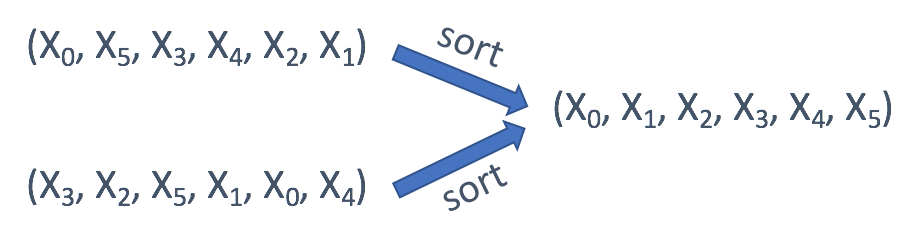• 如果只关心每个值的偏移量，通常是第一个值的偏移量，则可以使用偏移量作为键：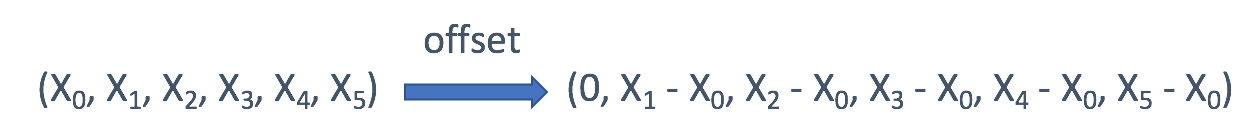• 在树中，可能会直接使用 TreeNode 作为键。 但在大多数情况下，采用子树的序列化表述可能是一个更好的主意。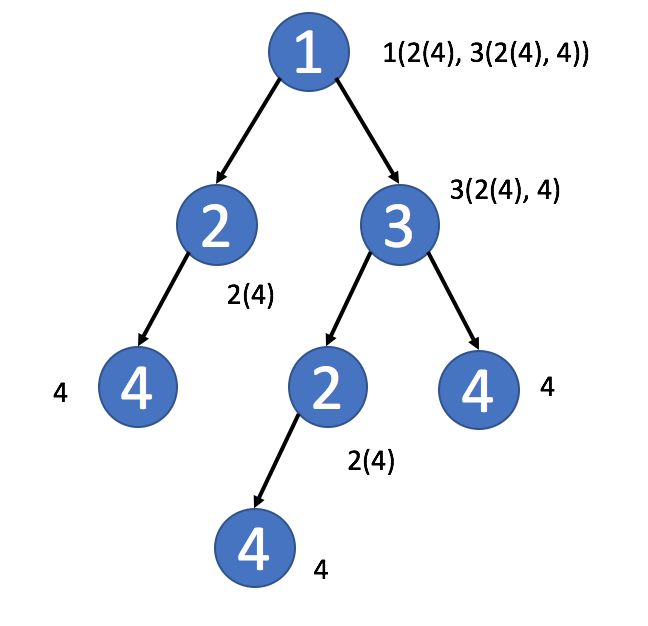• 在矩阵中，可以使用行索引或列索引作为键。
• 在数独中，可以将行索引和列索引组合来标识此元素属于哪个块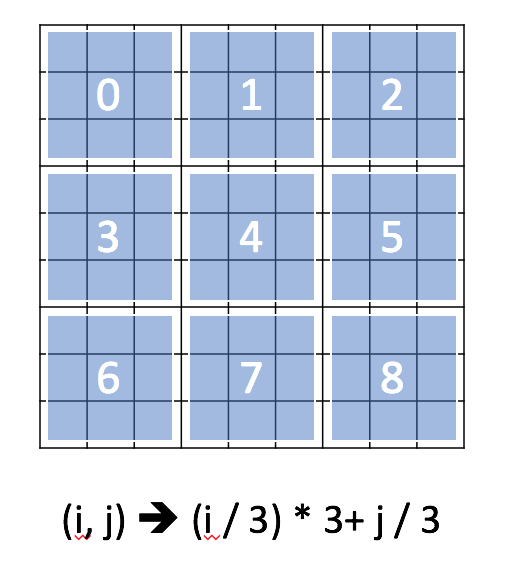• 有时，在矩阵中，您可能希望将值聚合在同一对角线中